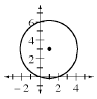### Home > A2C > Chapter Ch10 > Lesson 10.1.2 > Problem10-47

10-47.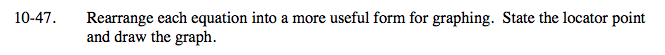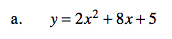Factor and complete the square.

y = 2(x2 + 4x) + 5

y = 2(x2 + 4x + 4) − 2(4) + 5

y = 2(x + 2)2 − 3

Locator point: (−2, −3)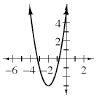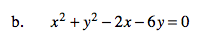Complete the square for both x and y.

(x − 1)2 + (y − 3)2 = 10

Locator point: (1, 3)